# 1st exampleIntroduction >
The structural frame
The construction
The reinforcement I
The reinforcement II
Quantity/Cost estimation
Detailing drawingsIntroduction >
Wind and Seismic Forces >
Structural model and Analysis
Modelling slabs
Slabs
Seismic behavour of frames
Appendix A
Appendix B
Appendix C
Appendix DIntroduction >
Materials
To be continued >

## 1st example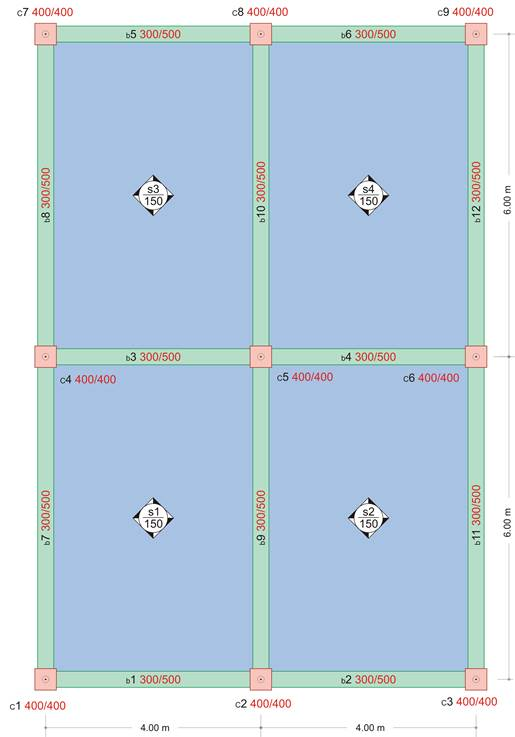(project <Β_422-1>)

The project deals with a simple one-storey building consisting of 9 columns, 12 beams and 4 slabs. The four slabs have identical dimensions of 4.0 mx6.0 m, thickness of 150 mm, covering load ge=1.0 kN/m2 and live load q=5.0 kN/m2. Concrete class: C30/37.

## 1st example: Shear force diagramsThe shear forces are equal to:

Direction x: Vxr=18.8Vx,erm=-33.3 [kN],         Direction y:Vyr=15.1Vy,erm=-29.0 [kN]

## 3D View of shear forces in x direction [Vx]## 3D View of shear forces in y direction [Vy]## 1st example: Bending moment diagramsThe bending moments are equal to:

Direction x: Mx=10.8, Mx,erm=-22.0 [kNm]                  Direction y: My=4.1,   My,erm=-16.2 [kNm]

## 3D View of bending moments in x direction [Mx]## 3D view of bending moments in y direction [My]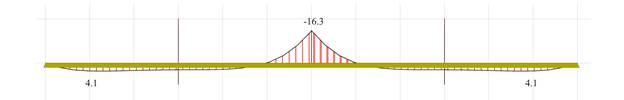## 1st example: Deflection diagramsΤhe maximum deflection of the slabs is equal to y=1.53 mm.

## The front view of deflection in x direction## The rear view of deflection in y direction## 3D distribution of shear forces [Vx]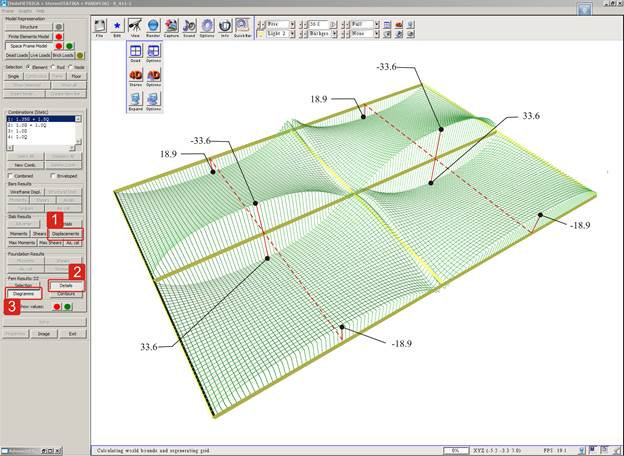## The front view of 3D distribution of shear forces [Vx]## The rear view of the 3D distribution of shear forces [Vx]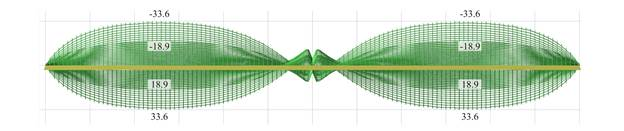## 3D distribution of shear forces [Vy]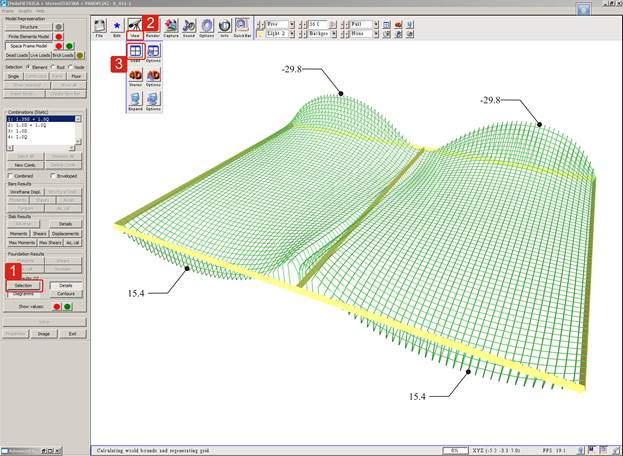## The front view of the 3D distribution of shear forces [Vy]## The rear view of the 3D distribution of shear forces [Vy]## 3D distribution of bending moments [Mx]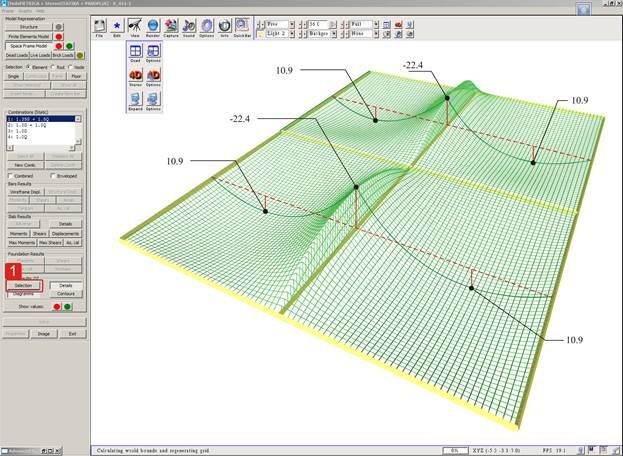## The front view of the 3D distribution of bending moments [Mx]## The rear view of the 3D distribution of bending moments [Mx]## 3D distribution of bending moments [My]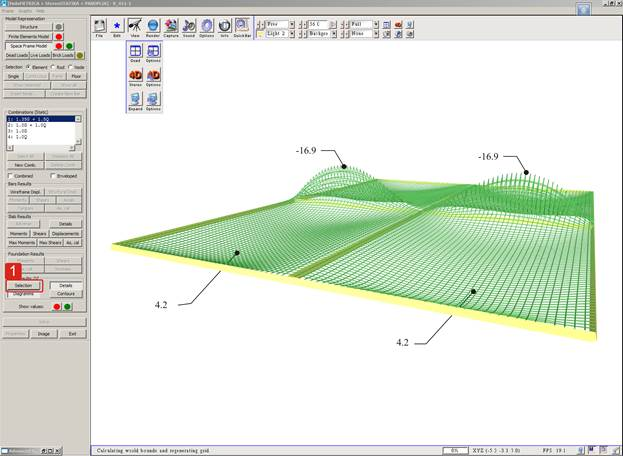## The front view of the 3D distribution of bending moments [My]## The rear view of the 3D distribution of bending moments [My]